# Basic Tutorial Lesson 5: Designing Basic Amplifier Circuits Using Op-Amp Devices

 Tutorial Project: Designing Basic Op-Amp Circuits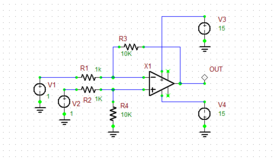Objective: In this project, you will build and examine a number of basic operational amplifier circuits. Concepts/Features: Operational Amplifier Live Simulation Live Parameters Gain Inverting Amplifier Non-Inverting Amplifier Minimum Version Required: All versions

## What You Will Learn

In this tutorial you will design a difference amplifier using the LM741 Op-Amp model. This Op-Amp can be replaced with any other Op-Amp part from various semiconductor manufacturers, like LM101, AD711, OP16, to name a few, or with RF.Spice's parameterized Op-Amp model. You will examine the operation of the amplifier using the "Live Parameters" feature of RF.Spice A/D. You will also investigate special cases of this circuit like inverting and non-inverting amplifiers and voltage follower.

The output voltage of the difference amplifier can be expressed in as a function of the two input voltages in the following form:

$V_{\text{out}} = \frac{ \left( R_1 + R_3 \right) R_4 }{\left( R_2 + R_4 \right) R_1} V_2 - \frac{R_3}{R_1} V_1$

subject to the constraint:

$R1 || R3 = R2 || R4$

If we set R1 = R2 and R3 = R4, the above equation is simplified to:

$V_{\text{out}} = \frac{ R_3 }{ R_1} \left( V_2 - V_1 \right)$

## Building the Difference Amplifier Circuit

The following is a list of parts needed for this project:

Part Name Part Type Part Value
V1 - V2 Voltage Source 1V
V3 - V4 Voltage Source 15V
R1 - R2 Resistor 1k
R3 - R4 Resistor 10k
X1 LM741 Op-Amp Defaults

Place the above parts on the schematic and connect them as shown on the figure. For the (-15V) negative DC supply source V4, you can either use a positive supply and flip it vertically (keyboard shortcut: Ctrl+Alt+F) as seen in the figure, or you can simply enter a DC voltage value of -15V. You can use a voltage probe marker (keyboard shortcut: ALt+L) to mark the output of the difference amplifier.

Your Op-Amp circuit is ready for simulation at this time. You will run a "Live Simulation" of this difference amplifier with Circuit Animation. In this tutorial lesson and the new two, you will use the "Live Parameters" feature of RF.Spice A/D. Go to the Toolbox side panel on the left of the screen and click on its "Parameters" tab. At the top of the panel, choose the "Some" option to answer the question "How many parameters?". In the parameter table, the parameters "v1.tran_dc" and "v2.tran_dc" represent the current voltages of the DC sources V1 and V2, both of which must be 1V at this time. Select the "v1.tran_dc" parameter, and type in the value "0.3" at the top of the panel. Make sure to press the "Down Arrow" or "Enter" button to make your change effective. Then select the "v2.tran_dc" parameter, and type in the value "0.2" for it.

Click the "Go/Run"button of the Main Toolbar to start the live simulation. Animate the circuit by clicking the "Show Voltage Text" button (with the letter V) on the Schematic Toolbar. You will see that an output voltage of about -1V on the schematic. While the simulation is still running, change the value of the "v2.tran_dc" parameter to "0.7". You will see that the output voltage now changes to about +4V. These results were expected because your difference amplifier has a gain equation given by:

$V_{\text{out}} = \left(\frac{ 10k }{ 1k }\right) \left( V_2 - V_1 \right) = 10 \left( V_2 - V_1 \right)$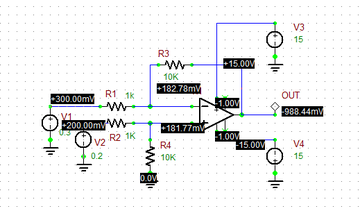Live voltage reading on the schematic for V1 = 0.3V and V2=0.2V.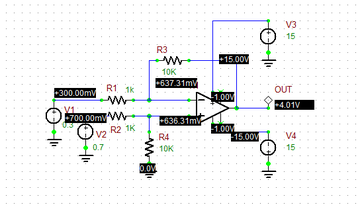Live voltage reading on the schematic for V1 = 0.3V and V2=0.7V.

## Testing an Inverting Amplifier Circuit

The above difference amplifier circuit is of a general form that can easily be reduced to the well-known Op-Amp Inverting Amplifier. All you have to do is to ground the "Non-Inverting" pin of the Op-Amp. This is equivalent to setting V2 = 0 and R2 = R4 = 0. The reduced circuit is shown below. Run a live simulation with V1 = 0.3V and animate the schematic. You will see an inverted output voltage of about -3V. The gain equation for the inverting amplifier is given by:

$V_{\text{out}} = -\left(\frac{ R3 }{ R1 }\right) V_{\text{in}} = -10 V_{\text{in}}$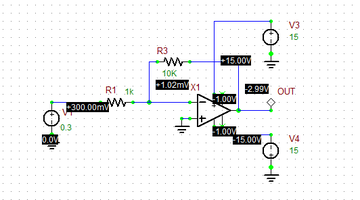Live voltage reading on the schematic of the inverting amplifier for V1 = 0.3V.

## Testing a Non-Inverting Amplifier Circuit

The original difference amplifier circuit can also easily be reduced to the well-known Op-Amp Non-Inverting Amplifier. All you have to do this time is to short-circuit the voltage source V1 and apply the voltage source V2 directly to the "Non-Inverting" pin of the Op-Amp. This is equivalent to setting V1 = 0, R2 = 0 and R4 = ∞. The reduced circuit is shown below. Run a live simulation with V1 = 0.2V and animate the schematic. You will see a non-inverted output voltage of about +2.2V. The gain equation for the non-inverting amplifier is given by:

$V_{\text{out}} = \left( 1 + \frac{ R3 }{ R1 }\right) V_{\text{in}} = 11 V_{\text{in}}$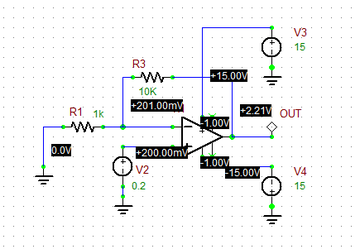Live voltage reading on the schematic of the non-inverting amplifier for V2 = 0.2V.

## Testing a Voltage Follower Circuit

The voltage follower is a special case of the Op-Amp Non-Inverting Amplifier with a unity gain. The figure below shows the Op-Amp voltage follower circuit. It can be used as an impedance buffer. This is equivalent to setting R1 = ∞ and R3 = 0. Run a live simulation with V1 = 0.2V and animate the schematic. You will see a non-inverted output voltage of about +0.2V, identical to the input voltage.Live voltage reading on the schematic of the voltage follower for V2 = 0.2V.